# Competitive Online Generalized Linear Regression Under Square Loss

Similarly to online linear regression, generalized linear regression models can be analyzed in the online framework. The logarithmic loss function is often used (see, e.g., Kakade and Ng, 2004). In this paper, the square loss function is considered. The Aggregating Algorithm is applied for the purpose of competing with the models. This problem does not appear to be analytically tractable. Therefore, the prediction algorithm is developed using the Markov chain Monte Carlo method.

Assume that the function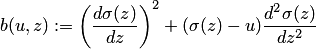is uniformly bounded: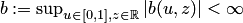.

Theorem. Let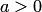. There exists a prediction strategy for Learner such that for every positive integer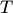, every sequence of outcomes of the length, and every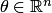, the cumulative square loss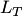of Learner satisfies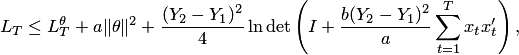where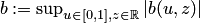. If in addition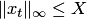for allthen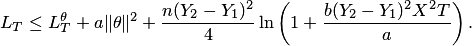It is shown that for linear regression, the bound is equivalent to the bound for the Aggregating Algorithm Regression (b=1).
For logistic regression,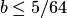.
For probit regression,.
For comlog regression,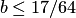.

Experiments are performed with the new algorithm on a toy data set and two real world ozone level data sets. The new algorithm is competitive with the Support Vector Machines with Gaussian kernel when applied either online and batch. It is worth noting that the class of the models covered by SVM is larger, and kernelization of the algorithm can improve the performance even further.

The Matlab package of the algorithms used is provided for public use.

### Bibliography

• Fedor Zhdanov and Vladimir Vovk. Competitive online generalized linear regression under square loss. In Proceedings of the European Conference on Machine Learning and Principles and Practice of Knowledge Discovery in Databases 2010, 2010.
• Sham M. Kakade and Andrew Y. Ng. Online bounds for Bayesian algorithms. In Proceedings of the 18th Annual Conference on Neural Information Processing Systems, 2004.